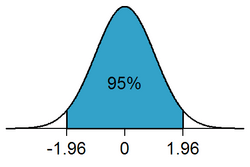# Statistical significance facts for kids

Kids Encyclopedia FactsIn a two-tailed test, the rejection region for a significance level of α=0.05 is partitioned to both ends of the sampling distribution and makes up 5% of the area under the curve (white areas).

Statistics uses variables to describe a measurement. Such a variable is called significant if the probability that its outcome was obtained by chance is less than a given value. Statistical hypothesis tests are used to check significance.

The concept of statistical significance was originated by Ronald Fisher when he developed statistical hypothesis testing, which he described as "tests of significance", in his 1925 publication, Statistical Methods for Research Workers. Fisher suggested a probability of one in twenty (0.05) as a convenient cutoff level to reject the null hypothesis. In their 1933 paper, Jerzy Neyman and Egon Pearson recommended that the significance level (e.g. 0.05), which they called α, be set ahead of time, before any data collection.

Despite his initial suggestion of 0.05 as a significance level, Fisher did not intend this cutoff value to be fixed, and in his 1956 publication Statistical methods and scientific inference he recommended that significant levels be set according to specific circumstances.In Spanish: Significación estadística para niños